# RBSE Maths Class 9 Chapter 14: Important Questions and Solutions

RBSE Maths Chapter 14 – Trigonometric Ratios of Acute Angles Class 9 Important questions and solutions are available here. The important questions and solutions of Chapter 14 provided at BYJU’S have detailed steps. Also, RBSE Class 9 solutions are given at BYJU’S to help the students in getting maximum marks in their exams and in improving their problem-solving skills.

Chapter 14 of the RBSE Class 9 Maths has formulas and identities of trigonometric ratios. Students will be introduced to finding the values of all the six trigonometric functions using a right triangle. Also, there exist problems in proving the trigonometric expressions using the relevant identities of trigonometry.

## RBSE Maths Chapter 14: Exercise 14.1 Textbook Important Questions and Solutions

Question 1: If in a ΔABC, ∠A = 90°, a = 25 cm, b = 7 cm, then find all the trigonometric ratios of ∠B and ∠C.

Solution:

Given,

∠A = 90°, a = 25 cm, b = 7 cm

Thus, BC = 25 cm and CA = 7 cm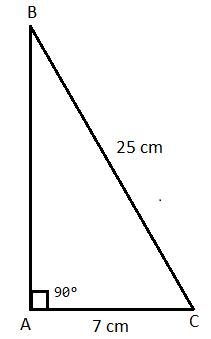In right triangle ABC,

AB2 + AC2 = BC2

AB2 = BC2 – AC2

AB = √(25)2 – (7)2

= √(625 – 49)

= √576

AB = 24

For acute angle B, trigonometric ratios are:

sin B = AC/BC = 7/25

cos B = AB/BC = 24/25

tan B = AC/AB = 7/24

cosec B = 1/sin B = 25/7

sec B = 1/cos B = 25/24

cot B = 1/tan B = 24/7

For acute angle C, trigonometric ratios are:

sin C = AB/BC = 24/25

cos C = AC/BC = 7/25

tan C = AB/AC = 24/7

cosec C = 1/sin C = 25/24

sec C = 1/cos C = 25/7

cot C = 1/tan C = 7/24

Question 2: If in a ΔABC, ∠B = 90°, a = 12 cm, b = 13 cm, then find all the trigonometric ratios of ∠A and ∠C.

Solution:

Given,

∠B = 90°, a = 12 cm, b = 13 cm

Thus, BC = 12 cm and AC = 13 cm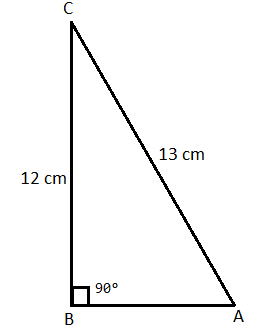In right triangle ABC,

AB2 + BC2 = AC2

AB2 = AC2 – BC2

= 132 – 122

= 169 – 144

= 25

AB = √25 = 5 cm

For acute angle A, all trigonometric ratios are:

sin A = BC/AC = 12/13

cos A = AB/AC = 5/13

tan A = BC/AB = 12/5

cosec A = 1/sin A = 13/12

sec A = 1/cos A = 13/5

cot A = 1/tan A = 5/12

For acute angle C, all trigonometric ratios are:cot A = 1/tan A = 5/12

sin C = AB/AC = 5/13

cos C = BC/AC = 12/13

tan C = AB/BC = 5/12

cosec C = 1/sin C = 13/5

sec C = 1/cos C = 13/12

cot C = 1/tan C = 12/5

Question 3: If tan A = √2 – 1, then prove that sin A cos A = 1/2√2.

Solution:

Given,

tan A = √2 – 1

Construct a triangle ABC in which ∠B = 90° and BC/AB = (√2 – 1)/1.

Let BC = (√2 – 1)k and AB = k, where k is proportionality constant.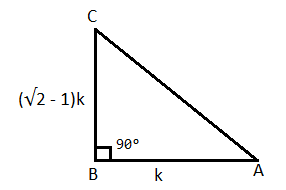In right triangle ABC,

AC2 = AB2 + BC2

= k2 + [(√2 – 1)k]2

= k2 + (2 – 2√2 + 1)k2

= k2 – 2√2k2 + 3k2

= (4 – 2√2)k2

AC = [√(4 – 2√2)]k

sin A = BC/AC = (√2 – 1)k/[√(4 – 2√2)]k = (√2 – 1)/√(4 – 2√2)

cos A = AB/AC = k/[(4 – 2√2)]k = 1/√(4 – 2√2)

LHS = sin A cos A

= [(√2 – 1)/√(4 – 2√2)] × [1/√(4 – 2√2)]

= (√2 – 1)/ (4 – 2√2)

= [(√2 – 1)/(4 – 2√2)] × [(4 + 2√2)/ (4 + 2√2)]

= [(√2 – 1) (4 + 2√2)}/ [(4)2 – (2√2)2]

= (4√2 + 4 – 4 – 2√2)/(16 – 8)

= (2√2)/8

= (2√2)/ (2√2)2

= 1/2√2

= RHS

Hence proved.

Question 4: If sin A = 1/3, then find the value of cos A cosec A + tan A sec A.

Solution:

Given,

sin A = ⅓ = Perpendicular/Hypotenuse

Construct a right triangle ABC in which, B is a right angle, BC = k, AC = 3k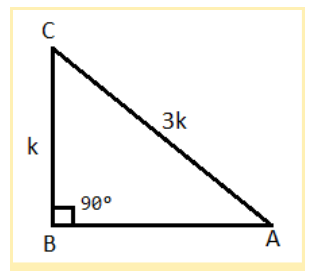In right triangle ABC,

AB2 + BC2 = AC2

AB2 = AC2 – BC2

= (3k)2 – k2

= 9k2 – k2

= 8k2

AB = √(8k2) = 2√2 k

cos A = AB/AC = 2√2k/3k = (2√2)/3

tan A = BC/AB = k/(2√2k) = 1/2√2

cosec A = 1/sin A = 3

sec A = 1/cos A = 3/2√2

cos A cosec A + tan A sec A = [(2√2)/3] × 3 + [1/(2√2)] × [3/(2√2)]

= 2√2 + [3/ (2√2)2]

= 2√2 + (⅜)

Question 5: If cos θ = 8/17, then find all the remaining trigonometric ratios.

Solution:

Given,

cos θ = 8/17 = Base/Hypotenuse

Construct a right triangle ABC in which, B is a right angle, AC = 17 k and AB = 8k.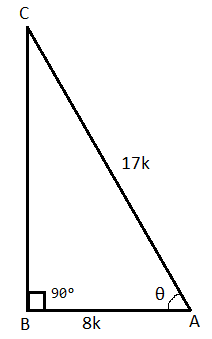In right triangle ABC,

AB2 + BC2 = AC2

BC2 = AC2 – AB2

= (17k)2 – (8k)2

= 289k2 – 64k2

= 225k2

BC = √(225k2) = 15k

sin θ = BC/AC = 15k/17k = 15/17

tan θ = BC/AB = 15/8

cosec θ = 1/sin θ = 17/15

sec θ = 1/cos θ = 17/8

cot θ = 1/tan θ = 8/15

Question 6: If cos A = 5/13, then find the value of cosec A/(cos A + cosec A).

Solution:

Given,

cos A = 5/13

Construct a right triangle ABC in which, B is a right angle, AC = 13k and AB = 5k.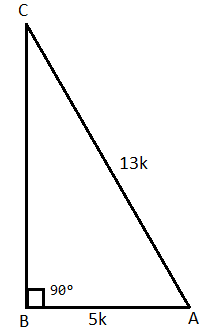In right triangle ABC,

AB2 + BC2 = AC2

BC2 = AC2 – AB2

= (13k)2 – (5k)2

= 169k2 – 25k2

= 144k2

BC = √(144k2) = 12k

cosec A = AC/BC = 13k/12k = 13/12

cosec A/ (cos A + cosec A) = (13/12)/ [(5/13) + (13/12)]

= (13/12)[(60 + 169)/156]

= (13/12) × (156/229)

= 169/229

Question 7: If 5 tan θ = 4, then find the value of (5 sin θ – 3 cos θ)/(sin θ + 2 cos θ).

Solution:

Given,

5 tan θ = 4

tan θ = ⅘ = Perpendicular/Base

Construct a triangle ABC in which B is a right angle and AB = 5k and BC = 4k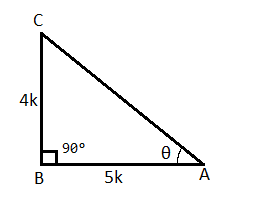AC2 = AB2 + BC2

= (5k)2 + (4k)2

= 25k2 + 16k2

= 41k2

AC = √(41k2) = √41 k

sin θ = BC/AC = 4k/√41 k = 4/√41

cos θ = AB/AC = 5k/√41 k = 5/√41

(5 sin θ – 3 cos θ)/ (sin θ + 2 cos θ) = [5(4/√41) – 3(5/√41)]/[(4/√41) + 2(5/√41)]

= [(20 – 15)/√41] × [(√41)/(4 + 10)]

= 5/14

Question 8: In ΔABC, ∠C = 90° and if cot A = √3 and cot B = 1/√3, then prove that sin A cos B + cos A sin B = 1.

Solution:

Given,

cot A = √3 and cot B = 1/√3

cot A = √3/1 = Base/Perpendicular

Construct a right triangle ABC in which B is right angle, AC =√3k and BC = k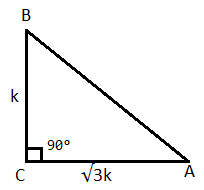AB2 = AC2 + BC2

= (√3k)2 + (k)2

= 3k2 + k2

= 4k2

AC = √(4k2) = 2k

sin A = BC/AB = k/2k = ½

cos A = AC/AB = √3k/2k = √3/2

sin B = AC/AB = √3k/2k = √3/2

cos B = BC/AB = k/2k = ½

LHS = sin A cos B + cos A sin B

= (½)(1/2) + (√3/2)(√3/2)

= (¼) + (¾)

= (1 + 3)/4

= 4/4

= 1 = RHS

Hence proved.

Question 9: If 16 cot A = 12, then find the value of (sin A + cos A)/(sin A – cos A).

Solution:

Given,

16 cot A = 12

cot A = 12/16 = 3/4

(sin A + cos A)/(sin A – cos A)

Dividing the numerator and denominator by sin A

= [(sin A/sin A) + (cos A/sin A)]/ [(sin A/sin A) – (cos A/sin A)]

= (1 + cot A)/(1 – cot A)

= [1 + (3/4)]/ [1 – (3/4)]

= [(4 + 3)/4]/ [(4 – 3)/4]

= 7

## RBSE Maths Chapter 14: Exercise 14.2 Textbook Important Questions and Solutions

Solve the following with the help of relation between trigonometric ratios.

Question 10: If cosec A = 5/4, then find the value of cot A, sin A and cos A.

Solution:

Given,

cosec A = 5/4

We know that,

cot2A + 1 = cosec2A

cot2A = cosec2A – 1

= (5/4)2 – 1

= (25/16) – 1

= (25 – 16)/16

= 9/16

cot A = √(9/16) = 3/4

sin A = 1/cosec A = 4/5

Now,

cot A = cos A/sin A

cos A = sin A cot A

= (4/5) × (3/4)

= 3/5

Therefore, cot A = 3/4, sin A = 4/5 and cos A = 3/5

Question 11: If cos B = 1/3, then find remaining trigonometric ratios.

Solution:

Given,

cos B = ⅓

We know that,

sin2B + cos2B = 1

sin2B = 1 – cos2B

= 1 – (1/3)2

= 1- (1/9)

= (9 – 1)/9

= 8/9

sin B = √(8/9) = 2√2/3

tan B = sin B/cos B

= (2√2/3)/ (1/3)

= 2√2

cosec B = 1/sin B = 3/2√2

sec B = 1/cos B = 3

cot B = 1/tan B = 1/2√2

Therefore, the remaining trigonometric ratios are:

sin B = 2√2/3, tan B = 2√2, cosec B = 3/2√2, sec B = 3, cot B = 1/2√2

Question 12: If sin A = 5/13, then find the value of cos A and tan A.

Solution:

Given,

sin A = 5/13

We know that,

sin2A + cos2A = 1

cos2A = 1 – sin2A

= 1 – (5/13)2

= 1- (25/169)

= (169 – 25)/169

= 144/169

cos A = √(144/169) = 12/13

tan A = sin A/cos A

= (5/13)/ (12/13)

= 5/12

Therefore, cos A = 12/13 and tan A = 5/12

Question 13: If tan A = 2, then find the value of sec A sin A + tan2A – cosec A.

Solution:

Given,

tan A = 2

We know that,

sec2A = 1 + tan2A

= 1 + (2)2

= 1 + 4

= 5

sec A = √5

cos A = 1/sec A = 1/√5

Now,

tan A = sin A/cos A

sin A = cos A tan A

= (1/√5) × (2)

= 2/√5

cosec A = 1/sin A = √5/2

sec A sin A + tan2A – cosec A = (√5) × (2/√5) + (2)2 – (√5/2)

= 2 + 4 – √5/2

= 6 – √5/2

= (12 – √5)/2

Question 14: If sin θ = 4/5, then find the value of (4 tan θ – 5 cos θ)/(sec θ + 4 cot θ).

Solution:

Given,

sin θ = 4/5

We know that,

sin2θ + cos2θ = 1

cos2θ = 1 – sin2θ

= 1 – (4/5)2

= 1 – (16/25)

= (25 – 16)/25

= 9/25

cos θ = √(9/25) = 3/5

tan θ = sin θ/cos θ

= (4/5)/ (3/5)

= 4/3

cot θ = 1/tan θ = 3/4

sec θ = 1/cos θ = 5/3

Now,

(4 tan θ – 5 cos θ)/ (sec θ + 4 cot θ) = [4 × (4/3) – 5 × (3/5)] / [(5/3) + 4 × (3/4)]

= [(16/3) – 3] / [(5/3) + 3]

= (16 – 9)/ (5 + 9)

= 7/14

= 1/2

Question 15: If sec θ = 2, then evaluate tan θ, cos θ and sin θ.

Solution:

Given,

sec θ = 2

We know that,

sec2θ = 1 + tan2θ

tan2θ = sec2θ – 1

= (2)2 – 1

= 4 – 1

= 3

tan θ = √3

cos θ = 1/sec θ = 1/2

Now,

tan θ = sin θ/cos θ

sin θ = tan θ cos θ

= (√3) × (1/2)

= √3/2

Therefore, tan θ = √3, cos θ = 1/2, and sin θ = √3/2

## RBSE Maths Chapter 14: Exercise 14.3 Textbook Important Questions and Solutions

Question 16: Prove that: cos θ.tan θ = sin θ

Solution:

LHS = cos θ.tan θ

= cos θ × (sin θ/cos θ)

= sin θ

= RHS

Therefore, cos θ.tan θ = sin θ

Hence proved.

Question 17: Prove that: (1 – sin2θ) tan2θ = sin2θ

Solution:

LHS = (1 – sin2θ) tan2θ

Using the identity sin2θ + cos2θ = 1,

= cos2θ × (sin2θ/cos2θ)

= sin2θ

= RHS

Therefore, (1 – sin2θ) tan2θ = sin2θ

Hence proved.

Question 18: Prove that: (cos2θ/sin θ) + sin θ = cosec θ

Solution:

LHS = (cos2θ/sin θ) + sin θ

= (cos2θ + sin2θ)/sin θ

Using the identity sin2θ + cos2θ = 1,

= 1/sin θ

= cosec θ

= RHS

Therefore, (cos2θ/sin θ) + sin θ = cosec θ

Hence proved.

Question 19: Prove that: (sin θ + cos θ)2 + (sin θ – cos θ)2 = 2

Solution:

LHS = (sin θ + cos θ)2 + (sin θ – cos θ)2

= sin2θ + cos2θ + 2 sin θ cos θ + sin2θ + cos2θ – 2 sin θ cos θ

= 2 sin2θ + 2 cos2θ

= 2(sin2θ + cos2θ)

Using the identity sin2θ + cos2θ = 1,

= 2(1)

= 2

= RHS

Therefore, (sin θ + cos θ)2 + (sin θ – cos θ)2 = 2

Hence proved.

Question 20: Prove that: cosec6θ – cot6θ = 1 + 3 cosec2θ cot2θ

Solution:

LHS = cosec6θ – cot6θ

= (cosec2θ)3 – (cot2θ)3

= (cosec2θ – cot2θ) [(cosec2θ)2 + cosec2θ cot2θ + (cot2θ)2]

Using the identity cot2θ + 1 = cosec2θ,

= (1)[cosec4θ + cot4θ + cosec2θ cot2θ]

= (cosec2θ – cot2θ)2 + 2 cosec2θ cot2θ + cosec2θ cot2θ

= (1)2 + 3 cosec2θ cot2θ

= 1 + 3 cosec2θ cot2θ

= RHS

Thus, cosec6θ – cot6θ = 1 + 3 cosec2θ cot2θ

Hence proved.

Question 21: Prove that: sin2θ cos θ + tan θ sin θ + cos3θ = sec θ

Solution:

LHS = sin2θ cos θ + tan θ sin θ + cos3θ

= (sin2θ cos θ + cos3θ) + tan θ sin θ

= cos θ × (sin2θ + cos2θ) + (sin θ/cos θ) × sin θ

Using the identity sin2θ + cos2θ = 1,

= cos θ × (1) + (sin2θ/cos θ)

= cos θ + (sin2θ/cos θ)

= (cos2θ + sin2θ)/cos θ

= 1/cos θ

= sec θ

= RHS

Thus, sin2θ cos θ + tan θ sin θ + cos3θ = sec θ

Hence proved.

Question 22: Prove that: [cos θ/ (1 – tan θ)] + [sin θ/ (1 – cot θ)] = sin θ + cos θ

Solution:

LHS = [cos θ/(1 – tan θ)] + [sin θ/(1 – cot θ)]

= [cos θ/ (1 – sin θ/cos θ)] + [sin θ/ (1 – cos θ/sin θ)]

= [cos2θ / (cos θ – sin θ)] + [sin2θ / (sin θ – cos θ)]

= [cos2θ / (cos θ – sin θ)] – [sin2θ / (cos θ – sin θ)]

= (cos2θ – sin2θ)/(cos θ – sin θ)

= [(cos θ + sin θ)(cos θ – sin θ)]/(cos θ – sin θ)

= cos θ + sin θ

= RHS

Thus, [cos θ/ (1 – tan θ)] + [sin θ/ (1 – cot θ)] = sin θ + cos θ

Hence proved.

Question 23: Prove that: [cosec θ/ (cosec θ – 1)] + [cosec θ/ (cosec θ + 1] = 2 sec2θ

Solution:

LHS = [cosec θ/ (cosec θ – 1)] + [cosec θ/ (cosec θ + 1]

= cosec θ [1/ (cosec θ – 1)] + [1/ (cosec θ + 1]

= cosec θ [(cosec θ + 1 + cosec θ – 1) / (cosec θ – 1)(cosec θ + 1)]

= cosec θ [2 cosec θ/ (cosec2θ – 12)]

= 2 cosec2θ/ (cosec2θ – 1)

Using the identity cot2θ + 1 = cosec2θ,

= 2 cosec2θ/cot2θ

= 2 × cosec2θ × tan2θ

= 2 × (1/ sin2θ) × (sin2θ/cos2θ)

= 2/cos2θ

= 2 sec2θ

= RHS

Thus, [cosec θ/ (cosec θ – 1)] + [cosec θ/ (cosec θ + 1] = 2 sec2θ

Hence proved.

Question 24: Prove that: sin θ/(1 – cos θ) = (1 + cos θ)/sin θ

Solution:

LHS = sin θ/ (1 – cos θ)

By rationalising the denominator,

= [sin θ/ (1 – cos θ)] [(1 + cos θ)/ (1 + cos θ)]

= sin θ(1 + cos θ)/ (12 – cos2θ)

= sin θ (1 + cos θ)/ (1 – cos2θ)

Using the identity sin2θ + cos2θ = 1,

= sin θ(1 + cos θ)/sin2θ

= (1 + cos θ)/sin θ

= RHS

Thus, sin θ/(1 – cos θ) = (1 + cos θ)/sin θ

Hence proved.

Question 25: Prove that: [sin θ/ (1 + cos θ)] + [(1 + cos θ)/sin θ] = 2 cosec θ

Solution:

LHS = [sin θ/ (1 + cos θ)] + [(1 + cos θ)/sin θ]

= [sin2θ + (1 + cos θ)2]/(1 + cos θ)sin θ

= [sin2θ + 1 + cos2θ + 2 cos θ]/(1 + cos θ) sin θ

Using the identity sin2θ + cos2θ = 1,

= (1 + 1 + 2 cos θ)/(1 + cos θ) sin θ

= (2 + 2 cos θ)/(1 + cos θ) sin θ

= 2(1 + cos θ)/(1 + cos θ) sin θ

= 2/sin θ

= 2 cosec θ

= RHS

Thus, [sin θ/ (1 + cos θ)] + [(1 + cos θ)/sin θ] = 2 cosec θ

Hence proved.

Question 26: Prove that:

$$\begin{array}{l}\sqrt{\frac{1-sin\theta}{1+sin\theta}}=\frac{1-sin\theta}{cos\theta}\end{array}$$

Solution:

$$\begin{array}{l}LHS=\sqrt{\frac{1-sin\theta}{1+sin\theta}}\end{array}$$

By rationalising the denominator,

$$\begin{array}{l}=\sqrt{\frac{1-sin\theta}{1+sin\theta}\times \frac{1-sin\theta}{1-sin\theta}}\\=\sqrt{\frac{(1-sin\theta)^2}{1^2-sin^2\theta}}\\=\sqrt{\frac{(1-sin\theta)^2}{cos^2\theta}}\\=\frac{1-sin\theta}{cos\theta}=RHS\end{array}$$

Thus,

$$\begin{array}{l}\sqrt{\frac{1-sin\theta}{1+sin\theta}}=\frac{1-sin\theta}{cos\theta}\end{array}$$

Hence proved.

Question 27: Prove that:

$$\begin{array}{l}\sqrt{\frac{sec\theta+1}{sec\theta-1}}=cot\theta+cosec\theta\end{array}$$

Solution:

$$\begin{array}{l}LHS = \sqrt{\frac{sec\theta+1}{sec\theta-1}}\end{array}$$

By rationalising the denominator,

$$\begin{array}{l}= \sqrt{\frac{sec\theta+1}{sec\theta-1}\times \frac{sec\theta+1}{sec\theta+1}}\\ =\sqrt{\frac{(sec\theta + 1)^2}{sec^2\theta-1^2}}\\=\sqrt{\frac{(sec\theta+1)2}{tan^2\theta}}\\=\frac{sec\theta+1}{tan\theta}\end{array}$$

$$\begin{array}{l}=\frac{sec\theta}{tan\theta}+\frac{1}{tan\theta}\end{array}$$

= sec θ cot θ + cot θ

= (1/cos θ) (cos θ/sin θ) + cot θ

= cosec θ + cot θ

= RHS

Thus,

$$\begin{array}{l}\sqrt{\frac{sec\theta+1}{sec\theta-1}}=cot\theta+cosec\theta\end{array}$$

Hence proved.

Question 28: Prove that: [√(cosec2θ – 1)]/cosec θ = cos θ

Solution:

LHS = [√(cosec2θ – 1)]/cosec θ

Using the identity cot2θ + 1 =cosec2θ,

= √(cot2θ)/cosec θ

= cot θ/cosec θ

= cot θ × sin θ

= (cos θ/sin θ) × sin θ

= cos θ

= RHS

Thus, [√(cosec2θ – 1)]/cosec θ = cos θ

Hence proved.

Question 29: Prove that: (1 + cot θ – cosec θ)(1 + tan θ + sec θ) = 2

Solution:

LHS = (1 + cot θ – cosec θ)(1 + tan θ + sec θ)

= [1 + (cos θ/sin θ) – (1/sin θ)] [1 + (sin θ/cos θ) + (1/cos θ)]

= [(sin θ + cos θ – 1)/ sin θ] [(cos θ + sin θ + 1)/ cos θ]

= [(sin θ + cos θ)2 – 12] / sin θ cos θ

= (sin2θ + cos2θ + 2 sin θ cos θ – 1)/ sin θ cos θ

Using the identity sin2θ + cos2θ = 1,

= (1 + 2 sin θ cos θ – 1)/sin θ cos θ

= (2 sin θ cos θ)/sin θ cos θ

= 2

= RHS

Thus, (1 + cot θ – cosec θ)(1 + tan θ + sec θ) = 2

Hence proved.

### RBSE Maths Chapter 14: Additional Important Questions and Solutions

Question 1: If √3 cos A = sin A, then the value of cot A is:

(A) √3

(B) 1

(C) 1/√3

(D) 2

Solution:

Given,

√3 cos A = sin A

cos A/sin A = 1/√3

cot A = 1/√3

Question 2: In given ΔABC, the value of cosec α is: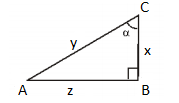(A) y/x

(B) y/z

(C) x/z

(D) x/y

Solution:

Given,

AB = z, BC = x and AC = y

cosec α = Hypotenuse / Opposite side to α

= AC/AB

= y/z

Therefore, cosec α = y/z

Question 3: The value of sin230° + cos230° is:

(A) 0

(B) 2

(C) 3

(D) 1

Solution:

sin230° + cos230°

Using the identity sin2A + cos2A = 1,

= 1

Question 4: The value of cosec255° – cot255° is:

(A) 1

(B) 2

(C) 3

(D) 0

Solution:

cosec255° – cot255°

Using the identity, cot2A + 1 = cosec2A (or cosec2A – cot2A = 1),

= 1

Question 5: If cot A = 20/21, then the value of cosec A is:

(A) 21/20

(B) 20/29

(C) 29/21

(D) 21/29

Solution:

Given,

cot A = 20/21

We know that, cosec2A = cot2A + 1

cosec2A = (20/21)2 + 1

= (400/441) + 1

= (400 + 441)/441

= 841/441

cosec A = √(841/441) = 29/21

Question 6: The value of (sec 40° + tan 40°)(sec 40° – tan 40°) is:

(A) -1

(B) 1

(C) cos 40°

(D) sin 40°

Solution:

(sec 40° + tan 40°)(sec 40° – tan 40°) = sec240° – tan240°

Using the identity 1 + tan2A = sec2A,

= 1

Question 7: The value of 1/(sin θ – tan θ) is:

(A) cot θ/(cos θ – 1)

(B) cot θ/(cot θ – cosec θ)

(C) cosec θ – cot θ

(D) cot θ

Solution:

1/(sin θ – tan θ)

= 1/ [sin θ – (1/ cot θ)]

= cot θ/(sin θ cot θ – 1)

= cot θ/ [sin θ × (cos θ/ sin θ) – 1]

= cot θ/(cos θ – 1)

Question 8: The value of (sec A – 1)/ (sec A + 1) is:

(A) (1 + cos A)/ (1 – cos A)

(B) (cos A – 1)/ (1 + cos A)

(C) (1 – cos A)/ (1 + cos A)

(D) (cos A – 1)/ (1 – cos A)

Solution:

(sec A – 1)/ (sec A + 1)

= [(1/cos A) – 1]/ [(1/cos A) + 1]

= [(1 – cos A)/cos A]/ [(1 + cos A)/cos A]

= [(1 – cos A) / cos A] × [cos A / (1 + cos A)]

= (1 – cos A)/(1 + cos A)

Question 9: The value of cot2θ – (1/sin2θ) is:

(A) 2

(B) 1

(C) 0

(D) -1

Solution:

cot2θ – (1/sin2θ)

= cot2θ – cosec2θ

= -1(cosec2θ – cot2θ)

Using the identity cot2A + 1 = cosec2A,

= -1 (1)

= -1

Question 10: f cos θ = 3/5, then evaluate (sin θ – cot θ)/2 tan θ.

Solution:

Given,

cos θ = 3/5

Using the identity sin2θ + cos2θ = 1,

sin2θ = 1 – cos2θ

= 1 – (3/5)2

= 1 – (9/25)

= (25 – 9)/25

= 16/25

sin θ = √(16/25) = 4/5

tan θ = sin θ/cos θ

= (4/5) / (3/5)

= (4/5) (5/3)

= 4/3

cot θ = 1/tan θ = 3/4

(sin θ – cot θ)/2 tan θ = [(4/5) – (3/4)]/ [2 × (4/3)]

= [(16 – 15)/20] / (8/3)

= (1/20) × (3/8)

= 3/160

Question 11: If cot θ = b/a, then evaluate (cos θ + sin θ)/ (cos θ – sin θ).

Solution:

Given,

cot θ = b/a

Now,

(cos θ + sin θ)/ (cos θ – sin θ)

Dividing the numerator and denominator by sin θ,

= [(cos θ/sin θ) + (sin θ/sin θ)] / [(cos θ/sin θ) – (sin θ/sin θ)]

= (cot θ + 1)/ (cot θ – 1)

Substituting cot θ = b/a,

= [(b/a) + 1]/ [(b/a) – 1]

= [(b + a)/a] / [(b – a)/a]

= (b + a)/(b – a)

Question 12: Prove that: √(sec2A + cosec2A) = tan A + cot A

Solution:

LHS = √(sec2A + cosec2A)

Using the identities: 1 + tan2A = sec2A and cot2A + 1 = cosec2A,

= √(1 + tan2A + 1 + cot2A)

= √(tan2A + cot2A + 2 × 1)

= √(tan2A + cot2A + 2 tan A cot A) (since tan A × cot A = 1)

= √(tan A + cot A)2

= tan A + cot A

= RHS

Thus, √(sec2A + cosec2A) = tan A + cot A

Hence proved.

Question 13: Prove that: (1 + sec θ)/sec θ = sin2θ/(1 – cos θ)

Solution:

LHS = (1 + sec θ)/sec θ

= (1/sec θ) + (sec θ/sec θ)

= cos θ + 1 ….(i)

RHS = sin2θ/(1 – cos θ)

By rationalising the denominator,

= [sin2θ/(1 – cos θ)] × [(1 + cos θ)/(1 + cos θ)]

= sin2θ(1 + cos θ) / (1 – cos2θ)

Using the identity sin2θ + cos2θ = 1,

= sin2θ(1 + cos θ)/sin2θ

= 1 + cos θ ….(ii)

From (i) and (ii),

(1 + sec θ)/sec θ = sin2θ/(1 – cos θ)

Hence proved.

Question 14: Prove that: (tan A + sec A – 1)/ (tan A – sec A + 1) = tan A + sec A

Solution:

LHS = (tan A + sec A – 1)/ (tan A – sec A + 1)

Using the identity 1 + tan2A = sec2A,

= [(tan A + sec A) – (sec2A – tan2A)] / (tan A – sec A + 1)

= [(tan A + sec A) – (sec A – tan A)(sec A + tan A)] / (tan A – sec A + 1)

= [(tan A + sec A)(1 – sec A + tan A)]/ (tan A – sec A + 1)

= tan A + sec A

= RHS

Hence proved.

Question 15: Prove that:

(i) tan2θ – cot2θ = sec2θ – cosec2θ

(ii) cos4θ – sin4θ = 1 – 2 sin2θ

Solution:

(i) tan2θ – cot2θ = sec2θ – cosec2θ

LHS = tan2θ – cot2θ

Using the identities: 1 + tan2A = sec2A, 1 + cot2A = cosec2A,

= (sec2θ – 1) – (cosec2θ – 1)

= sec2θ – 1 – cosec2θ + 1

= sec2θ – cosec2θ

= RHS

Hence proved.

(ii) cos4θ – sin4θ = 1 – 2 sin2θ

LHS = cos4θ – sin4θ

= (cos2θ)2 – (sin2θ)2

= (cos2θ + sin2θ)(cos2θ – sin2θ)

Using the identity sin2θ + cos2θ = 1,

= (1)[(1 – sin2θ) – sin2θ]

= 1 – 2 sin2θ

= RHS

Hence proved.

Question 16: Prove that: √[(cosec2θ – 1)/ cosec2θ] = cos θ

Solution:

LHS = √[(cosec2θ – 1)/ cosec2θ]

Using the identity 1 + cot2θ = cosec2θ,

= √(cot2θ/ cosec2θ)

= cot θ/cosec θ

= (cos θ/sin θ) × sin θ

= cos θ

= RHS

Hence proved.

Question 17: If sin A = 3/5, then find the value of cos A and cot A.

Solution:

Given,

sin A = 3/5

We know that,

sin2A + cos2A = 1

cos2A = 1 – sin2A

= 1 – (3/5)2

= 1- (9/25)

= (25 – 9)/25

= 16/25

cos A = √(16/25) = 4/5

cot A = cos A/sin A

= (4/5)/ (3/5)

= 4/3

Therefore, cos A = 4/5 and cot A = 4/3